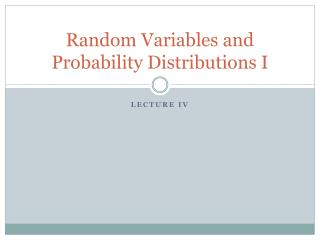# Random Variables and Probability Distributions I - PowerPoint PPT PresentationDownload PresentationRandom Variables and Probability Distributions I

Random Variables and Probability Distributions IDownload Presentation## Random Variables and Probability Distributions I

- - - - - - - - - - - - - - - - - - - - - - - - - - - E N D - - - - - - - - - - - - - - - - - - - - - - - - - - -
##### Presentation Transcript

1. Conditional Probability and Independence • In order to define the concept of a conditional probability it is necessary to discuss joint probabilities and marginal probabilities. • A joint probability is the probability of two random events. For example, consider drawing two cards from the deck of cards. There are 52x51=2,652 different combinations of the first two cards from the deck. • The marginal probability is overall probability of a single event or the probability of drawing a given card.

2. The conditional probability of an event is the probability of that event given that some other event has occurred. • In the textbook, what is the probability of the die being a one if you know that the face number is odd? (1/3). • However, note that if you know that the role of the die is a one, that the probability of the role being odd is 1. • Axioms of Conditional Probability: • P(A|B) ≥ 0 for any event A. • P(A|B) = 1 for any event A B. • If {Ai  B}, i=1,2,3,… are mutually exclusive, then • If B  H, B  G and P(G)  0 then

3. Theorem 2.4.1: for any pair of events A and B such that P(B)  0. • Theorem 2.4.2 (Bayes Theorem): Let Events A1, A2, … An be mutually exclusive such that P(A1A2  … An)=1 and P(Ai)>0 for each i. Let E be an arbitrary event such that P(E)>0. Then

4. Another manifestation of this theorem is from the joint distribution function: • The bottom equality reduces the marginal probability of event E • This yields a friendlier version of Bayes theorem based on the ratio between the joint and marginal distribution function:

5. Statistical independence is when the probability of one random variable is independent of the probability of another random variable. • Definition 2.4.1: Events A, B and C are said to be independent if P(A) = P(A|B). • Definition 2.4.2: Events A, B, and C are said to be mutually independent if the following equalities hold:

6. Basic Concept of Random Variables Definition 1.4.1: A random variable is a function from a sample space S into the real numbers. In this way a random variable is an abstraction

7. The probability function (or measure) is then defined based on that random variable:

8. Definition of a Random Variable Definition 3.1.1. A random variable is a variable that takes values according to a certain probability. Definition 3.1.2. A random variable is a real-valued function defined over a sample space.

9. Discrete Random Variables Definition 3.2.1. A discrete random variable is a variable that takes a countable number of real numbers with certain probability. Definition 3.2.2. A bivariate discrete random variable is a variable that takes a countable number of points on the plane with certain probability

10. In a bivariate distribution, the marginal distribution is the distribution of one variable unconditioned on the outcome of the other variable

11. Applying Bayes Theorem Definition 3.2.3. Discrete random variables are said to be independent if the event (X=xi), and the event (Y=yj) are independent for all i,j. That is to say, P(X=xi,Y=yj)= P(X=xi)P(Y=yj).

12. Uncorrelated Binomial

13. Conditional Probabilties

14. Uncorrelated Discrete Normal

15. Conditional Probabilities

16. Correlated Discrete Normal

17. Conditional Probabilities

18. Theorem 3.2.1. Discrete Random variables X and Y with the probability distribution given in table 3.1 are independent if and only if every row is proportional to any other row, or, equivalently, every column is proportional to any other column.

19. Multivariate Random Variables Definition 3.2.4. A T-variate random variable is a variable that takes a countable number of points on the T-dimensional Euclidean space with certain probabilities.

20. Univariate Continuous Random Variables Definition 3.3.1. If there is a nonnegative function f(x) defined over the whole line such that for any x1, x2 satisfying x1x2, then X is a continuous random variable and f(x) is called its density function

21. By axiom 2, the total area under the density function must equal 1: The simplest example of a continuous random variable is the uniform distribution:

22. It is obvious that

23. Definition 3.3.2. Let X have density f(x). The conditional density of X given aXb, denoted by f(x| aXb), is defined by

24. Definition 3.3.3. Let X have the density f(x) and let S be a subset of the real line such that P(XS)>0. Then the conditional density of X given XS, denoted by f(x|S), is defined by

25. Common Univariate Distributions Uniform Distribution

26. Gamma Distribution

27. Normal Distribution

28. Beta Distribution